# Ratios in Chemistry

Stoichiometry is a part of chemistry that establishes the quantitative relationships between the reagents and products of reactions. This technique is then repeated with the newly discovered value and the process continues. No matter how complicated a problem might seem there will only be three values to interact with: a known value for a component of a reaction (reagent or a product), an unknown value of the other thing (product or other reagent), and a third number that represents the exact relationship (ratio) between the two units. The relationship number is called a stoichiometric ratio, or a conversion factor. Thus, being able to form ratios and setting up equations that use them to convert amounts of one component into the amount of the other is the central skill in stoichiometry. As such, the first section of this tutorial will only deal with how to derive ratios and use them mathematically. The subsequent sections will examine the same mathematics within the context of chemistry.

A simple way to think about ratios is a comparison of two values. Specifically, they compare how many times one value can fit inside the other value. For example, if you had a bowl of 8 red candies and 4 yellow candies there would be two red candies for every yellow candy so the ratio of red candy to yellow candy would be two. There are three ways to express ratios; as two values separated by a colon, as a fraction, or as the quotient of the first value divided by the second value. So, the ratio of red to yellow candy could be expressed as 8:4, 8/4, or 2. However, for the purposes of stoichiometry, it is most convenient to express ratios as fractions. Also, in science, it’s usually a good idea to express the units as well as the values which would look like this:

8 red:4 yellow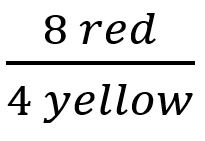2 red/yellow

Depending on the preference of the writer, the ratios might also be expressed in their simplest forms:

2 red:1 yellow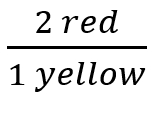2 red/yellow

If the relationship between two units is constant, then the ratio of those units will also remain constant even as the values change. For example, if a car can drive a fixed 25 miles for every 1 gallon of gasoline, then the ratio of miles/gallons, sometimes called mileage, would always be 25 miles/gallon regardless of if the driver drove 1 mile or 100 miles. This means that the driver can use one ratio to easily calculate the amount of gas required to drive any distance by dividing the distance by the mileage. People use ratios like this intuitively in their daily life, however it can be intimidating to try to represent in formal mathematics, or when the units are unfamiliar. Let’s expand the example above and try to understand what is happening mathematically.

Imagine that the owner of previous car wanted to find how many gallons of gas it would take to drive 100 miles. People who drive often might be able to easily find the answer, but it is worthwhile to examine how the problem is set up because in chemistry there will be no way to solve these problems unless the equations are set up correctly. When doing unit conversions, it is important to start with the known value in this question the given value is 100 miles. Next multiply the known value by a conversion factor. In this case our conversion factor is the mileage: 25miles/gallon, however, it is not useful in this form. When converting units, the equation must be set up so that so that the original units cancel out and you are left with a number that only has the desired units. If the value 100 miles is multiplied by the conversion factor 25 miles/hour the result is nonsensical: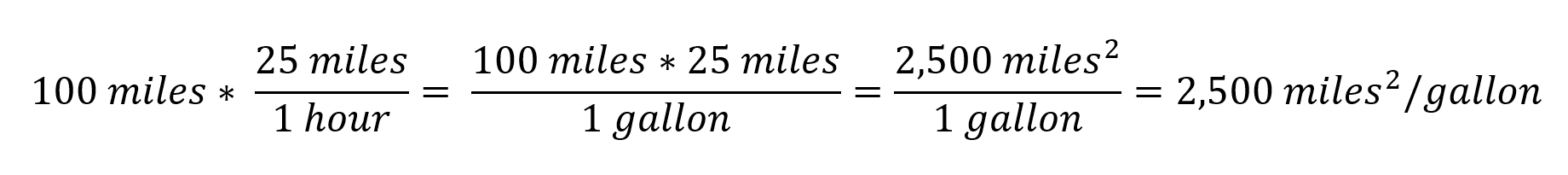The solution to this equation doesn’t have meaning in this context, and  doesn’t look like the desired units of only gallons. This is a strong indication that the conversion factor was used incorrectly.  To set up the equation correctly the miles in the starting value, and the miles in the conversion factor must cancel out. Instead of using the ratio miles/gallon as the conversion factor the correct equation uses the reciprocal, gallons/mile, to convert to gallons.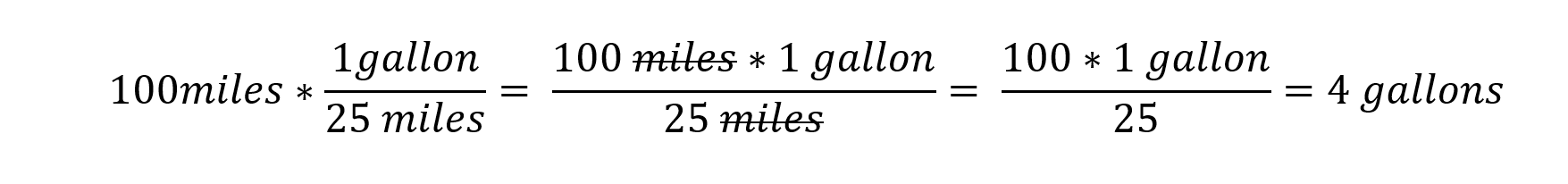Notice that when the equation is correctly set up the units of the known value is always expressed in the denominator (bottom part) of the conversion factor. This allows the units to cancel out and is a simple way to tell if you are using the correct conversion factor. Multiplying by the reciprocal of the mileage has the same functional effect as dividing by the mileage, however, it is highly encouraged to write in the form of a known value multiplied by a fraction, as shown above. It is much easier to understand how the values are interacting with each other and to go back and correct any mistakes when the equation is written out in this longer form. Below is an example problem showing how unit conversions can be used in situations with unusual units. Try to solve the problem yourself before reading the solution.

Jon is throwing a party and wants to know how much pizza he needs to buy for his guests. He figures that for every 12 guests he will need to buy 5 large pizzas. If there are 36 guests coming over how many pizzas does Jon need?

The first step is to figure out what units are being asked for and what units are given. This will determine the structure of the equation. In the question the number of guests is given, and it asks for the number of pizzas.  So, the first thing written in the equation will be 36 guests, and result at the end should be given in number of pizzas.

The second step is to determine which conversion factor to multiply the number of guests by. The problem gives the ratio 12 guests:5 pizzas, but in this fraction the number of guests is expressed in the top if the fraction, and it needs to be expressed in the bottom to cancel out the guests in the known value. In this case the reciprocal should be used.

Now that the equation is set up the final step is to multiply the known value by the correct conversion factor: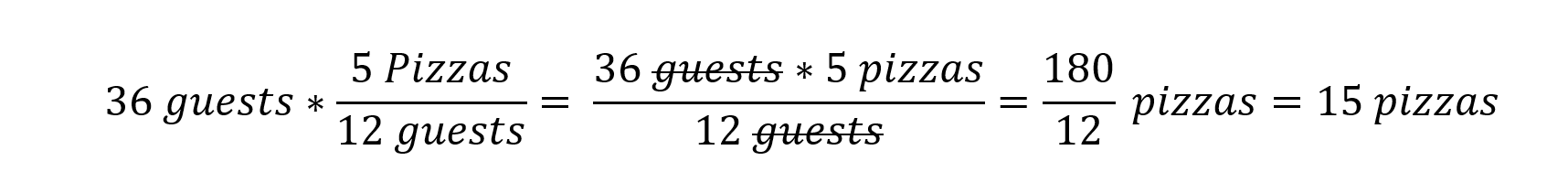While working the problem, the units of the known value cancel out and the number at the end is expressed in the units of the desired value. This is a good indication that the solution is probably correct. If the product had different units than what was expected it would be necessary to go back and re-examine the mathematics, as well as how the equation was set up.

The ability to set up and solve unit conversions as described in this article is the only knowledge of mathematics that is required for stoichiometry.  However, the units in the conversion factors will not be miles/gallon, or pizza/guest. Instead, the conversion factors will be derived from units given in chemical equations. To perform the appropriate conversions, it will be necessary to understand the anatomy of a chemical equation. This will be the subject of the next part in this series.